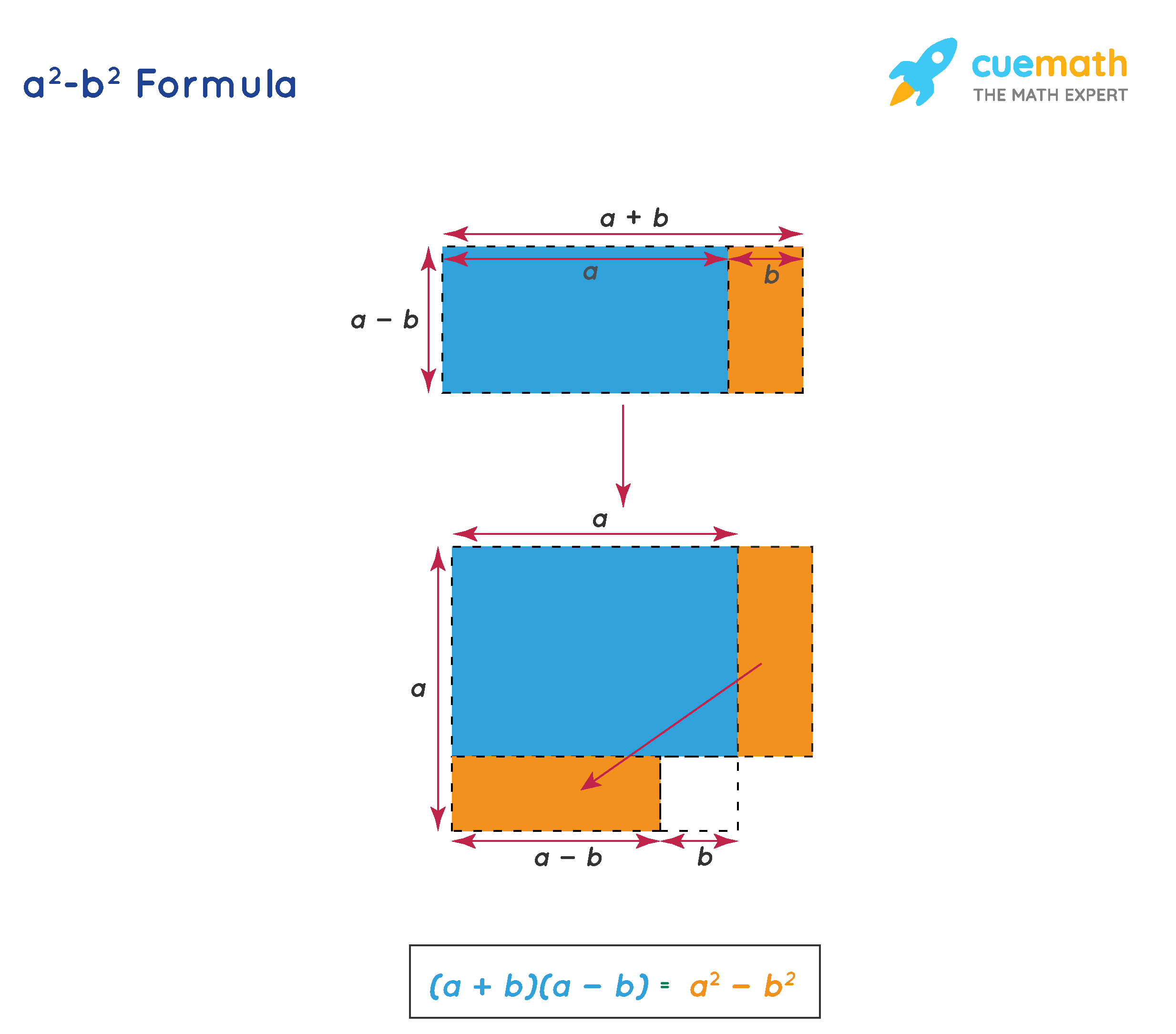# a^2-b^2 Formula

The a- b2 formula is also known as "the difference of squares formula".

## What is a^2-b^2 Formula?

The a- b2 formula is:

a- b= (a - b) (a + b)

If you would like to verify this, you can just multiply (a - b) (a + b) and see whether you get a2 - b2

You can understand this formula geometrically using the following figure:## Solved Examples Using a^2-b^2 Formula

### Find the value of 1062 - 62.

Solution: To find: 1002 - 62.

Let us assume that a = 100 and b = 6.

We will substitute these in the a- b2 formula.

a- b= (a - b) (a + b)

1062 - 62 = (106 - 6) (106 + 6)

= (100) (112)

= 11200

### Factorize the expression 25x2 - 64.

Solution: To factorize: 25x2 - 64.

We will use the a- b2 formula to factorize this.

We can write the given expression as

25x2 - 64 = (5x)2 - 82

We will substitute a = 5x and b = 8 in the formula of a2 - b2

a- b= (a - b) (a + b)

(5x)2 - 82 = (5x - 8) (5x + 8)